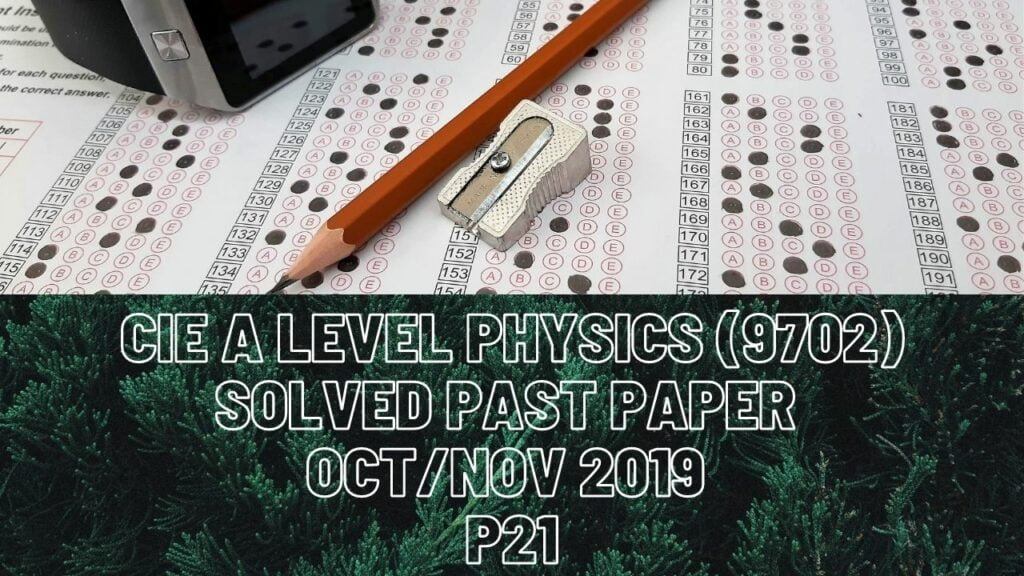A Level Physics### CIE A Level Physics Solved Past Paper Oct/Nov 2019 P21

1 bii) For absolute uncertainty of value T, you first need to have its % uncertainty. For % uncertainty, you will have to add up % uncertainties of all quantities being used in the formula. For T, those are mass, m, and spring constant, k BUT before you take their sum, you must multiply each % uncertainty with the number of times that quantity is being used in the formula. Like m and k are being taken root of so multiply their % uncertainties with 1/2 and finally take their sum. At last, you have to convert that % uncertainty to absolute uncertainty by dividing it by 100% and multiply that to the value of T.

3a) Mass=density*volume where density of air being propelled by one propeller is 1.2 kg/m^3 and volume of air being propelled downwards in interval of 3s is what we must calculate first. We are to assume that propelled air travels 7.6m every second in a cylinder which has a diameter of 16cm (0.16m). The volume it covers in a second is (22/7*(0.16/2)^2*7.6)=0.153m^3 so in 3s it must cover a volume of 0.4584m^3. Now mass=1.2*0.4584=0.55kg of air.

ci) Since each propeller is at fixed position meaning that its at equilibrium. Its upward force must be equal to its downward force. The downward force exerted by each propeller on 0.55kg of air is 1.4N so the same amount of force must act on each propeller too.

d) Force on both propellers(makes up most of aircraft’s weight)= mass of aircraft*acceleration